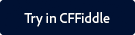Note:

You can find the CFFiddle demo of this function and other spreadsheet functions as part of a project that is shared with you.

Click the button below to launch CFFiddle.To copy the project in your workspace in CFFiddle, follow the steps below:

2. Navigate to the project in the left pane.
3. Once you make some changes in any cfm in the project, a pop up displays asking you to save the project.
4. Give the project a suitable name and click Save.

Description

Specifies the formula for an Excel spreadsheet object cell.

Returns

Does not return a value.

Category

Microsoft Office Integration

History

ColdFusion 9: Added the function.

Parameters

Parameter

Description

The Excel spreadsheet object to which to add the comment.

formula

A string containing the formula.

row

The row number of the cell to which to add the formula.

column

The column number of the cell to which to add the formula.

Usage

This function replaces any existing value, including specific entered values.

Example

The following line sets the formula for the cell at row 2 column 11 to be the sum of the cells in the column's rows 1 through 12.The following example sets a cell formula, and gets the cell formula and value.

 //Create a new Excel spreadsheet object. theSheet=SpreadsheetNew(); //Set the values of column 3 rows 1-10 to the row number. for (i=1; i<= 10; i=i+1) SpreadsheetSetCellValue(theSheet,i,i,3); //Set the fomula for the cell in row 11 column 3 to be the sum of //Columns 1-10. SpreadsheetSetCellFormula(theSheet,"SUM(C1:C10)",11,3); //Get the formula from the Excel spreadsheet object. theValue=SpreadsheetGetCellFormula(theSheet,11,3); //Get the value of row 11 column 5 from the Excel spreadsheet object. theValue=SpreadsheetGetCellValue(theSheet,11,3); Row 11, Column 3 value: #SpreadsheetGetCellValue(theSheet,11,3)#
Row 11, Column 3 formula: #SpreadsheetGetCellFormula(theSheet,11,3)#Twitter™ and Facebook posts are not covered under the terms of Creative Commons.